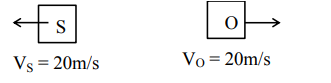# A source and a detector move away from each other in absence of`
Question:

A source and a detector move away from each other in absence of wind with a speed of $20 \mathrm{~m} / \mathrm{s}$ with respect to the ground. If the detector detects a frequency of $1800 \mathrm{~Hz}$ of the sound coming from the source, then the original frequency of source considering speed of sound in air $340 \mathrm{~m} / \mathrm{s}$ will be $\mathrm{Hz}$

Solution:$f^{\prime}=f\left(\frac{C-V_{0}}{C+V_{s}}\right)$

$1800=f\left(\frac{340-20}{340+20}\right)$

$f=2025 \mathrm{~Hz}$

Ans. 2025